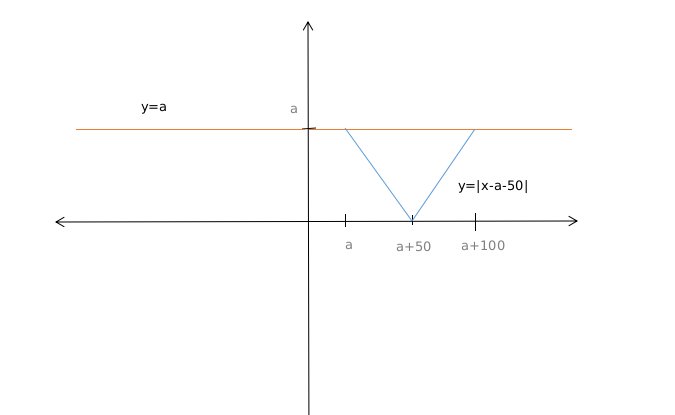Question 48

# Let $$0 \leq a \leq x \leq 100$$ and $$f(x) = \mid x - a \mid + \mid x - 100 \mid + \mid x - a - 50\mid$$. Then the maximum value of f(x) becomes 100 when a is equal to

Solution

x>=a, so |x-a| = x-a

x<100, so |x-100| = 100-x

f(x) = (x-a) + (100-x) + |x-a-50| =100

or, |x-a-50| = aFrom the graph we can can see that when x=a then

|x-a-50|=a

or, a= 50

Similarly when x=a+100

|x-a-50|=a

or, a= 50

So value of a is 50 when f(x) is 100.

### View Video Solution

• All Quant CAT Formulas and shortcuts PDF
• 30+ CAT previous papers with solutions PDF